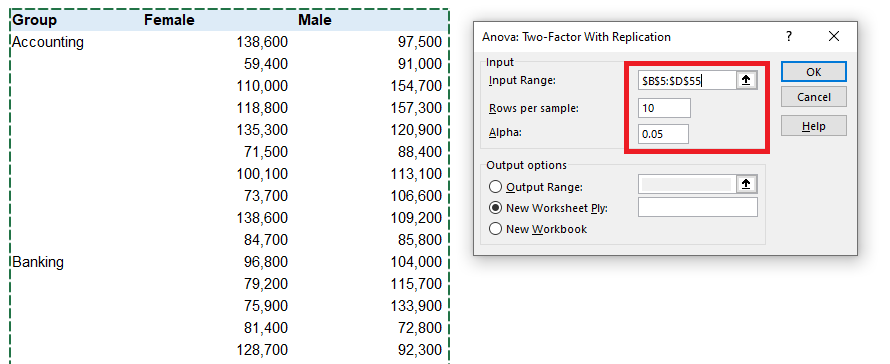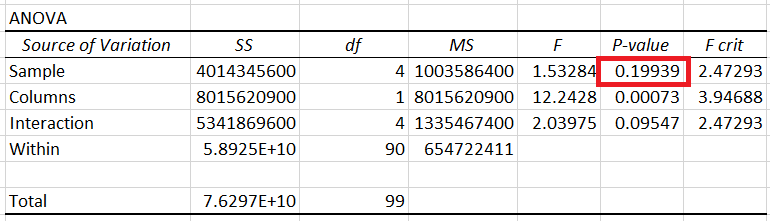## How to Run a Two-Way Analysis of Variance (ANOVA) in Excel

Recently, we looked at how to Perform a One-Way Analysis of Variance in Excel. In today’s article, we will take that a step further and a look at a Two-Factor ANOVA.

The Two-Way Analysis of Variance (ANOVA) is a statistical test to evaluate the difference between the means of more than two groups.

It is also known as a Factorial ANOVA with two factors. We use the model when we have one measurement variable and two nominal variables, also known as factors or main effects. To employ this analysis, we need to have measurements for all possible combinations of the nominal values.

The method estimates how the mean of quantitative variable changes in connection to the different levels (positions) of two categorical values. In other words, this form of ANOVA helps analyze how to independent variables combinedly influence a dependent variable from a statistical point of view. We can also employ the method to evaluate whether the two independent factors have a significant interaction effect.

## How Does ANOVA Work?

To run the Two-Way ANOVA model, we need to collect data on the quantitative dependent variable at different combinations (levels) of two independent categorical variables.

Each categorical value should have finite possible values or factor levels. They divide the dependent variable’s observations into groups for each combination of the categories.

The quantitative metric should be one for which we can take measures and calculate a mean (average). Observations need to be of sufficient quantity so that we can calculate an average for each combination of the levels in the categorical metrics.

The Analysis of Variance model relies on an F-test to check statistical significance. The F-test is a comparison test, which compares the variance in each group’s mean value to the overall variance in the dependent variable.

If the variance within the groups is smaller than the overall variance, the F-value will be higher, meaning the observed difference is most likely real, and not due to chance.

ANOVA is a test of hypotheses that we use to evaluate the differences between group means. The model uses sample data to infer the characteristics of the entire population.

## Replication and Interaction

We usually run the Two-Way ANOVA model with replication, meaning that there is more than one observation for each combination of the independent variables. We can also perform the analysis without replication, where we only have a single measurement for each arrangement of the factors. However, this is less informative as we can’t test for Interaction, and often have to assume there is none.

The interaction effect indicates that one factor impacts the relationship between the dependent variable and the other categorical variable.

Based on that, there are two types of ANOVA tests we can run for the categorical variables – with and without Interaction.

If we are analyzing a model without Interaction, we test the following two null hypotheses (H0):

1. No difference in the group means at any level of the first independent variable;
2. No difference in the group means at any level of the second independent variable.

In case you end up testing a model with Interaction, we add one additional null hypothesis:

1. The impact of one independent variable does not affect the impact of the other independent variable.

If the interaction term in a Two-Way ANOVA model turns out to be significant, the most common analysis approach is to evaluate each of the two factors separately, with a One-Way ANOVA.

It is crucial to remember that when we run the model without replication, we cannot test the Interaction. If we identify a significant difference in the means for one of the factors, there’s no way to tell if this difference is consistent for other values of the second factor.

## Assumptions

When we perform a Two-Way ANOVA analysis, our data should meet certain assumptions, typical for a parametric test of differences.

Our data should exhibit homoscedasticity, or homogeneity of the variance. This means the variation around the mean value for each group has to be similar between groups. The observations also have to be independent and unique and have an equal standard deviation. The data for the dependent variable should follow a bell curve or be normally distributed.

## Two-Way ANOVA in Excel

The Analysis of Variance is a well-known method within the fields of finance and valuation. As the most widely-available tool in these industries is Excel, we can find the model within the in-built Analysis Tool Pack within the software. However, keep in mind that the available model in Excel is not as robust as in specialized statistical software.

One of the most prominent limitations of Excel is that it can only take balanced designs meaning each group has the same number of observations. Running a Two-Way ANOVA with an unbalanced design is significantly more complex and challenging from a computational perspective, and you will need some statistics software to perform this.

To perform a two-factor analysis of variance in Excel, first, we need to format our data properly. Let’s look at an example to understand the concept better. We are looking at the categorical values Gender and Industry, and the dependent variable Salary. We have obtained information on the annual Salary for ten people for each group made by all combinations of factor levels. We prepare our data as a matrix with one factor on the rows and one on the columns:Once our data is formatted in this way, and we have ensured to include an equal quantity of observations for each combination of the two categories, we can run the ANOVA model.

Go to the Data tab and open Data Analysis.Note, if you don’t see it there, go into the Add-Ins options and enable Analysis ToolPak.From the Data Analysis menu, we will select Anova: Two-Factor With Replication.The following screen asks us to input some details. First, select the input range. Then remember to properly include the Rows per sample number, which is the number of observations within each combination of factors. For our data, this is ten. You can leave the Alpha at 0.05, which is usually the value we use in financial analysis and modeling.Hit OK, and Excel will generate a new worksheet containing all the relevant statistical information for the Two-Way ANOVA model.

Let’s start by evaluating whether the Industry independent variable has a statistically significant effect on the Salary dependent variable.The P-value is 0.19939. As this exceeds the 0.05 Alpha level, the result has no statistical significance. In other words, we can conclude that the Industry has no significant impact on the Salary.

Next, let us look at Gender and assess whether it has a notable impact.The P-value for this factor is less than 0.05. Because of that, we consider Gender to be statistically significant. In other words, we can note a substantial difference in Salary between Females and Males.

The last F-test we will look at is the one for Interaction.The P-value is 0.09547, which means the result is not statistically significant. There is no significant interaction between Industry and Gender.

In conclusion, we know that Industry doesn’t influence Salary materially, while Gender has a substantial impact on the Salary variable. Therefore, we expect the Female and Male groups to differ from each other.Looking at the mean for each group, we see that the average Salary in the Female group is 100 thousand, while for the Male group it is almost 20% more, sitting at 118 thousand. This comparison is not enough to assess whether the difference is statistically significant, but it’s an excellent way to get a general idea about the data.

## Conclusion

In the end, the Two-Way ANOVA model identifies the impact of two categorical variables on a dependent variable. It is a powerful analytical tool that we can run in Excel and any specialized statistics software. However, it is essential to remember it’s underlying assumptions and the limitations that they create.

Please, show your support by sharing the article with colleagues and friends. Also, don’t forget to subscribe to our newsletter (no spam, ever!) and download the data for the ANOVA model below.## FCCA, FMVA

Hi! I am a finance professional with 10+ years of experience in audit, controlling, reporting, financial analysis and modeling. I am excited to delve deep into specifics of various industries, where I can identify the best solutions for clients I work with.

In my spare time, I am into skiing, hiking and running. I am also active on Instagram and YouTube, where I try different ways to express my creative side.